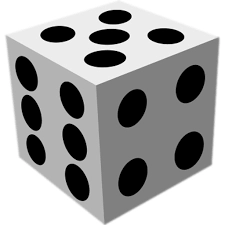# A fair dice

A fair six-sided dice is rolled. What is the probability that the first number rolled is greater than 1 and the second number rolled is odd? Type your answer as a fraction in the simplest form.

p =  5/12

### Step-by-step explanation:Did you find an error or inaccuracy? Feel free to write us. Thank you!

Tips for related online calculators
Need help calculating sum, simplifying, or multiplying fractions? Try our fraction calculator.
Would you like to compute the count of combinations?